SOLVING MAGNETOSPHERIC ACCELERATION, RECONNECTION AND TURBULENCE

REAL TIME
SPACE WEATHER

 STATUS INDEX 68.18 PCP 2.8 PKP 1.0 KP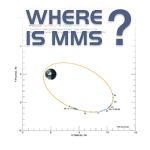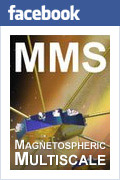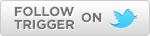SPACE WEATHER : REAL TIME : INTERPRETATION GUIDE

Space Weather Dials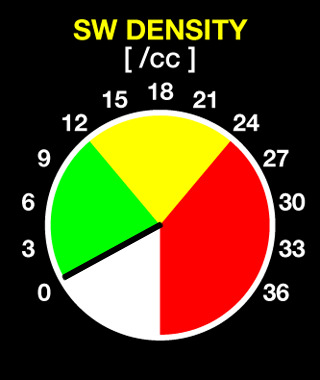Solar Wind Density: (Measured)This quantity is the number of solar wind protons per unit volume as measured by the ACE Solar Wind Electron Proton Alpha Monitor (SWEPAM).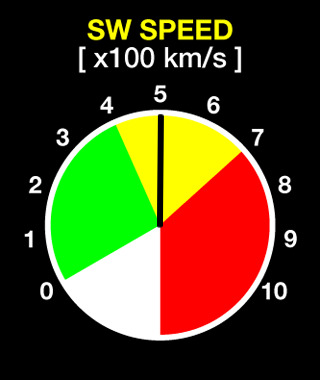Solar Wind Speed: (Measured)This quantity is the average (bulk) speed of solar wind protons as measured by ACE/SWEPAM. This is the solar wind speed just as the bulk speed of air molecules is the wind speed we know here on the surface of the Earth.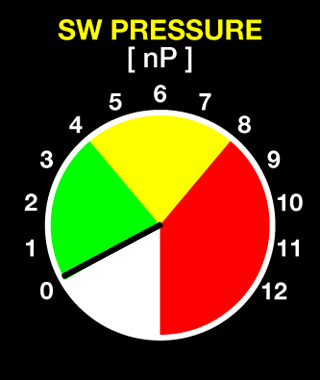Solar Wind Pressure: (Derived)This quantity is the solar wind ram pressure, the force per unit area required to stop the solar wind flow. This is similar in concept to the force a surface wind exerts on a sail. The solar wind ram pressure depends on the solar wind speed and density.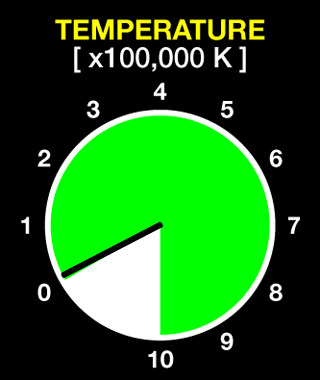Solar Wind Temperature: (Measured)This quantity is the temperature of protons in the solar wind. It is measured by ACE/SWEPAM.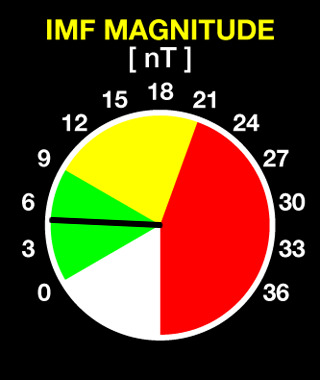Interplanetary Magnetic Field Magnitude: (Measured)This quantity is the strength of the interplanetary magnetic field (IMF) as measured by the ACE Magnetometer (MAG).Interplanetary Magnetic Field Polar Angle: (Derived)This quantity is the angle between the IMF and the geomagnetic axis. When the IMF is southward, antiparallel fields near the magnetospheric subsolar point allow merging between the IMF and geomagnetic fields. This process increases the transport of solar wind mass, momentum, and energy into the Earth's magnetosphere. This process can also open the magnetosphere to solar energetic particle radiation. In severe conditions this radiation can threaten high altitude aircraft in high latitude and polar regions. Under less severe conditions this radiation can still threaten polar orbiting spacecraft. This quantity depends on IMF components measured by ACE/MAG.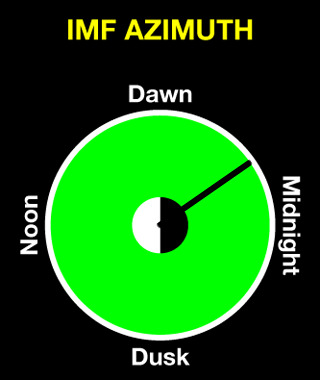Interplanetary Magnetic Field Azimuth: (Derived)This quantity is the direction of the IMF perpendicular to the geomagnetic axis. This affects the details of solar wind-magnetosphere interactions; however, this is of tertiary importance compared to the IMF magnitude and polar angle. This quantity also depends on IMF components measured by ACE/MAG.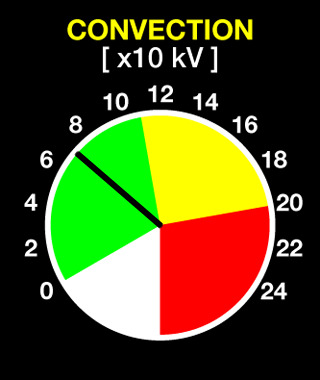Voltage Across the Polar Cap / Convection Potential: (Derived)This quantity measures the solar wind energy input to the magnetosphere that drives magnetospheric convection. It appears as an electric potential imposed across the polar ionosphere. The quantity shown here is an estimate of the asymptotic convection potential based on ACE/SWEPAM and ACE/MAG measurements as well as the work of Boyle, et al. (Journal of Geophysical Research 102, 111, 1997.) This estimate is asymptotic because it does not account for the time delays such as those imposed by friction between the ionosphere and the neutral atmosphere.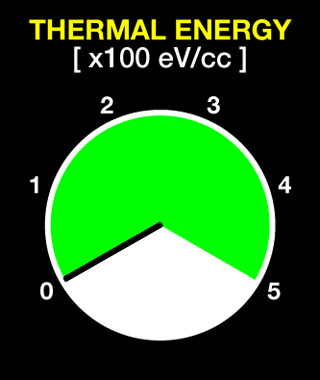Thermal Energy Density: (Derived)This quantity is the heat content of the solar wind. It takes into account both solar wind density and temperature and can be translated into solar wind thermal pressure. This quantity is generally less important than either the solar wind ram pressure or the solar wind (IMF) magnetic pressure.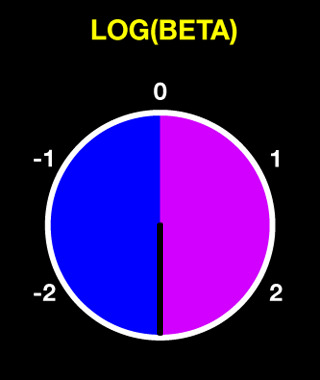Log[Beta]: (Derived)Beta is the ratio between the thermal and magnetic energy densities in the solar wind; this ratio controls whether particle thermal processes or magnetic processes dominate the behavior of the plasma. The base-10 logarithm of Beta is shown on the dial. The blue portion of the dial shows when magnetic processes govern solar wind structures; the purple portion shows when thermal processes govern these structures.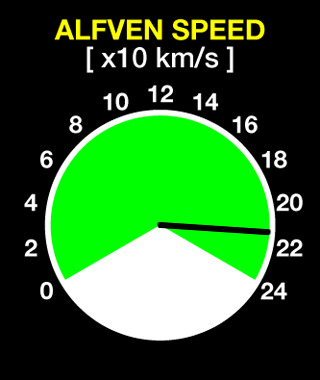Alfven Speed: (Derived) This quantity is the propagation speed of shear Alfven (intermediate mode) magnetohydrodynamic waves in the solar wind.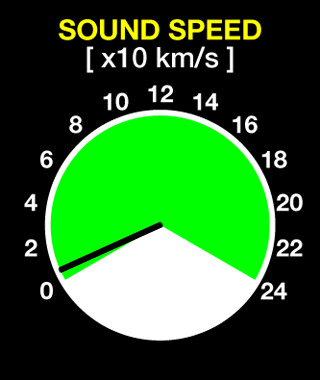Sound Speed: (Derived) This quantity is the propagation speed of gasdynamic (sound) waves in the solar wind. Although collision rates are generally so low in the solar wind that classic sound waves do not travel effectively, this quantity is necessary for calculating the propagation speeds of compressional Alfven (fast and slow mode) magnetohydrodynamic waves in the solar wind. The magnetohydrodynamic waves together allow the use of gasdynamic approximations to describe portions of the solar wind-magnetosphere interaction.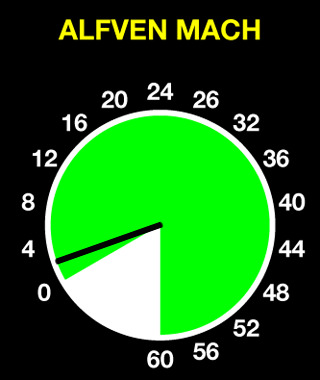Alfven Mach Number: (Derived)This quantity is the ratio between the solar wind speed and the Alfven speed. This normally controls the type of bow shock required to divert the solar wind around the magnetosphere. This bow shock is similar to the shock in front of a supersonic airplane that generates a "sonic boom" when the shock passes an observer.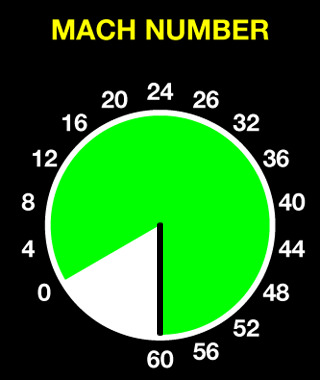Mach Number: (Derived)This quantity is the classic Mach number, the ratio between the solar wind speed and the gasdynamic sound speed. This quantity controls the bow shock when the solar wind plasma is in a high-Beta (Log[Beta]>0) state.